# Numbers up to 2-Digits

Numbers up to 2-Digits
Go back to  'Number Systems'

In this video, join hands with Rita and Joel in counting sticks on a farm. Watch out for the trick that Rita uses, when there are more than 10 sticks.

 1 Concept Video on Numbers 2 Introduction to Numbers up to 2-Digits 3 The Big Idea: Numbers up to 2-Digits 4 Two Digit Number 5 How to Find Number of Digits in a Number? 6 How is it Important? 7 Challenging Questions 8 Common Mistakes 9 Solved Examples 10 Practice Questions 11 Important Notes 12 Maths Olympiad Sample Papers 13 Frequently Asked Questions (FAQs)

We at Cuemath believe that Math is a life skill. Our Math Experts focus on the “Why” behind the “What.” Students can explore from a huge range of interactive worksheets, visuals, simulations, practice tests, and more to understand a concept in depth.

Book a FREE trial class today! and experience Cuemath’s LIVE Online Class with your child.

## Concept Video on Numbers

Watch this video on how numbers are introduced to kids the Cuemath Way!

Let us understand the Why? behind the What!

## Introduction to Numbers up to 2-Digits

We begin counting numbers on the fingers of our hands at a very young age, and then go on to using supercomputers to crunch numbers which are very large.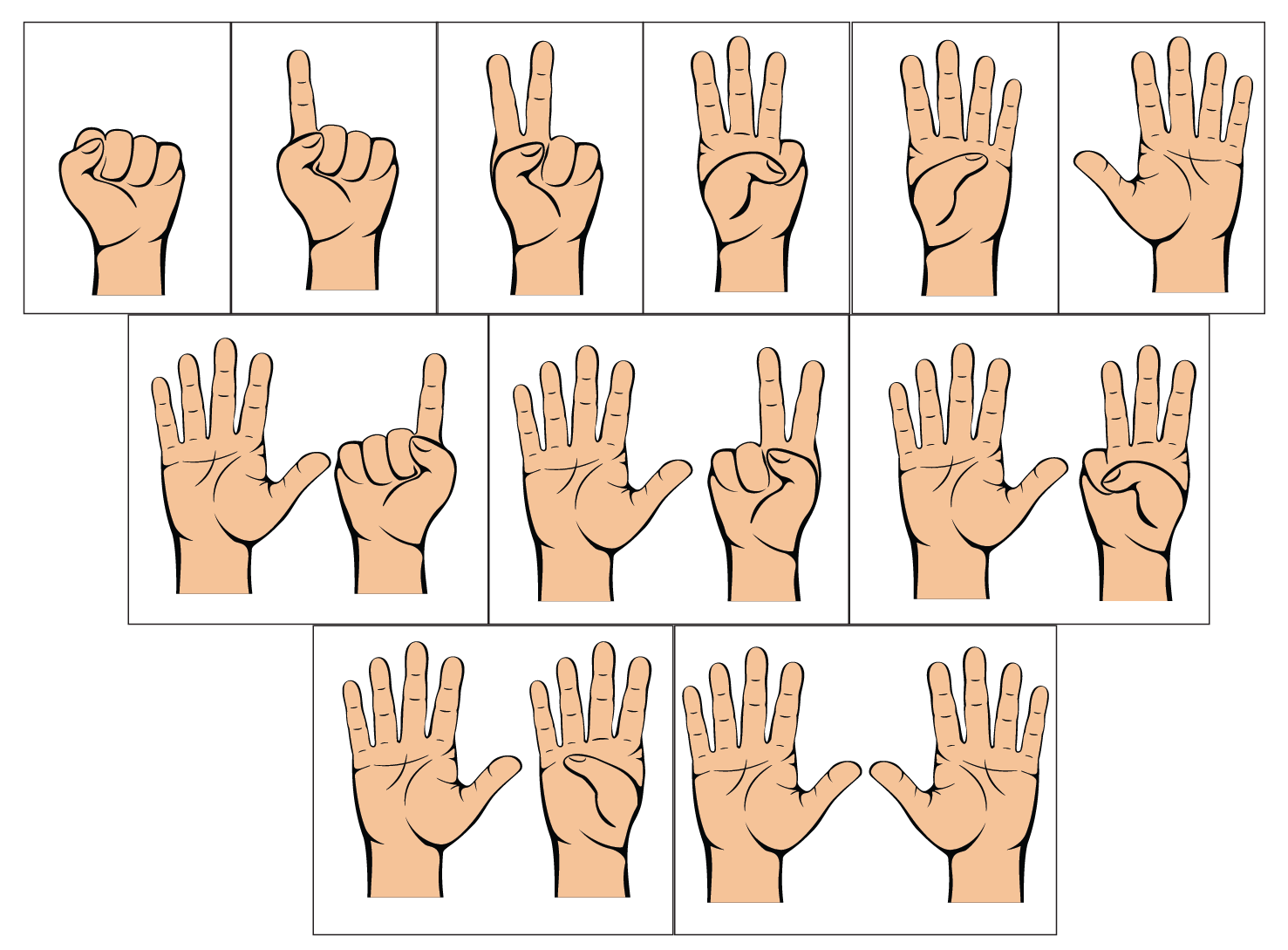Whatever be the count of digits of a number, the correct placement of each digits is most important.

Today we will discuss 2-digit numbers, but before that, let us familiarize ourselves with single digit numbers.

There are 10 single digit numbers -$$0,1,2,3,4,5,6,7,8$$ and $$9$$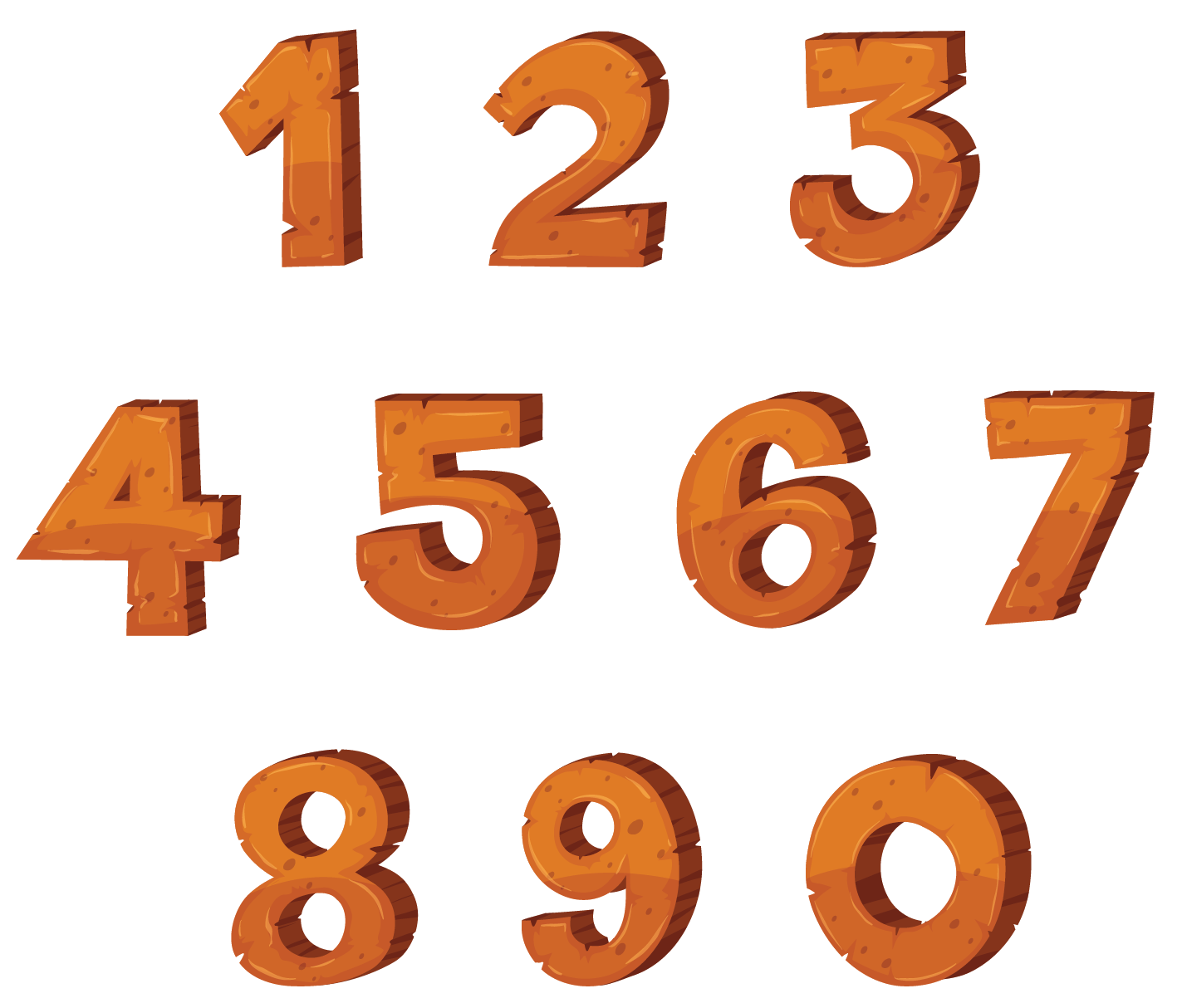All the double-digit or 2-digit numbers we will discuss are combinations of any two of nine of these ten numbers.

Yes, 0 is not always counted, and we will come to that later.

Watch this simulation to see how a 2-digit number is represented in blocks and rods.

10 of the blocks put together forms a rod.

10 Ones is equal to 1 Tens.

## The Big Idea: Numbers up to 2-Digits

### A Simple Idea: The Place Value of Numbers

Every number which has more than 1 digit has to have the different digits described by their place values.

The place values we use are units, tens, hundreds, thousands, ten thousands, and so on.

You will notice that each of the consecutive place values is ten times the previous one.

When we limit ourselves to 2-digit numbers, therefore, there are only two place values in play – the Units place and the Tens place.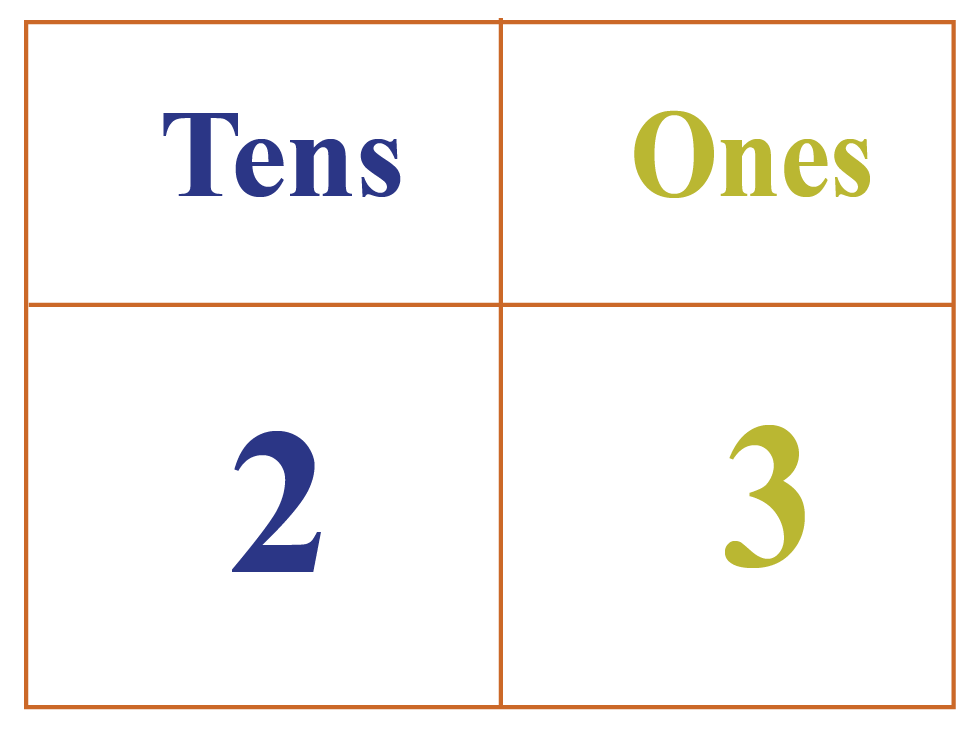Let us say you buy ice cream from a shop, and you have 5 friends coming over for lunch.

Including your parents, you need 5 $$+$$ You $$+$$ Parents = 8 ice creams.

You can go to the shop and buy 8 units of ice creams.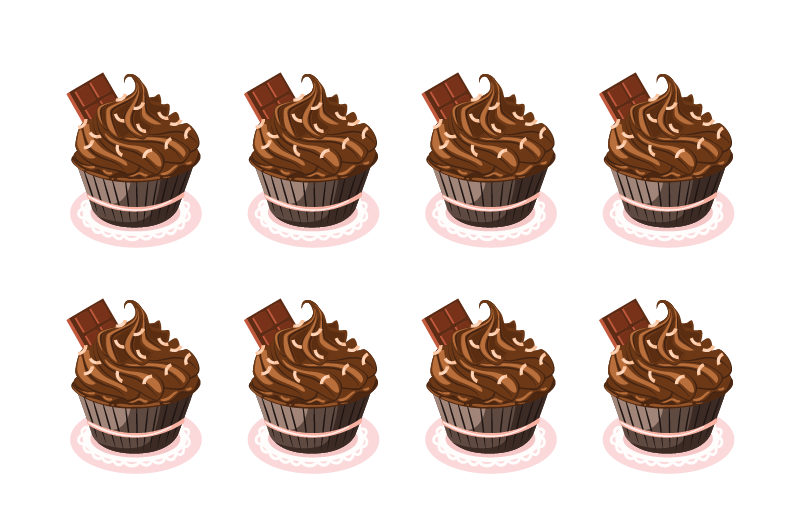If you invite your whole class home when you are ready to leave school, you have 33 friends $$+$$ you $$+$$ your parents = 36 ice creams.

So, you will take 3 tubs to give you the equivalent of 30 ice creams.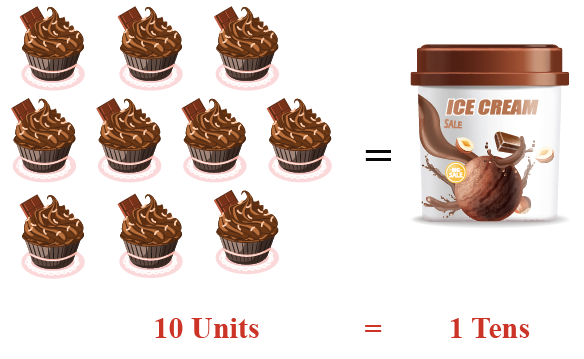In addition, you will take 6 ice cream cups, making it 36 ice creams in all.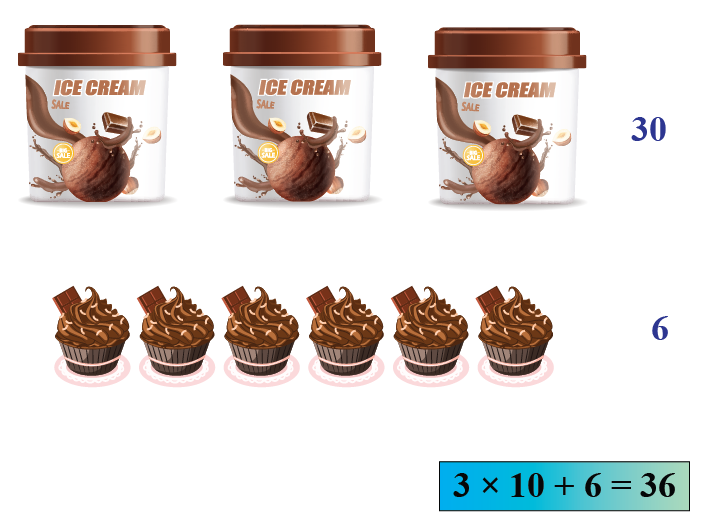This is exactly what is done when we create two digit numbers.

### Assigning Place Values

So, every set of ten units becomes a number with a place value of ten, just like the shopkeeper made 1 tub by combining 10 ice cream cups.

Let us look at 3 sets of numbers to understand the conversion of units to a combination of units and tens:

\begin{align} &{\text{33 units: 3 Tens + 3 Units}}\\ &{\text{8 units: 1 Ten + 8 Units}}\\ &{\text{27 units: 2 Tens + 3 Units}} \end{align}

I am sure you have been able to make out that only the first one is correct.

The second one has the tens place wrong, and the third one has the units place wrong.

This is what the right combinations would be for both sets:

\begin{align} { 3 \text { Tens } + 3 \text { Units } \\= ( 3 \times 10 ) + ( 3 \times 1 ) \\= 30 + 3 = 33 } \\ { 1 \text { Ten } + 8 \text { Units } \\= ( 1 \times 10 ) + ( 8 \times 1 ) \\= 10 + 8 = 18 } \\ { \text { 2 Tens } + 3 \text { Units }\\ = ( 2 \times 10 ) + ( 3 \times 1 ) \\= 20 + 3 = 23 } \end{align}

\begin{align}& 33 \text { units: } \\&( 3 \times 10 ) + ( 3 \times 1 ) \\&= 30 + 3 = 33 \\ &8 \text { units: }\\ &( 0 \times 10 ) + ( 8 \times 1 ) \\&= 0 + 8 = 8 \\ & 27 \text { units: }\\& ( 2 \times 10 ) + ( 7 \times 1 )\\& = 20 + 7 = 27 \end{align}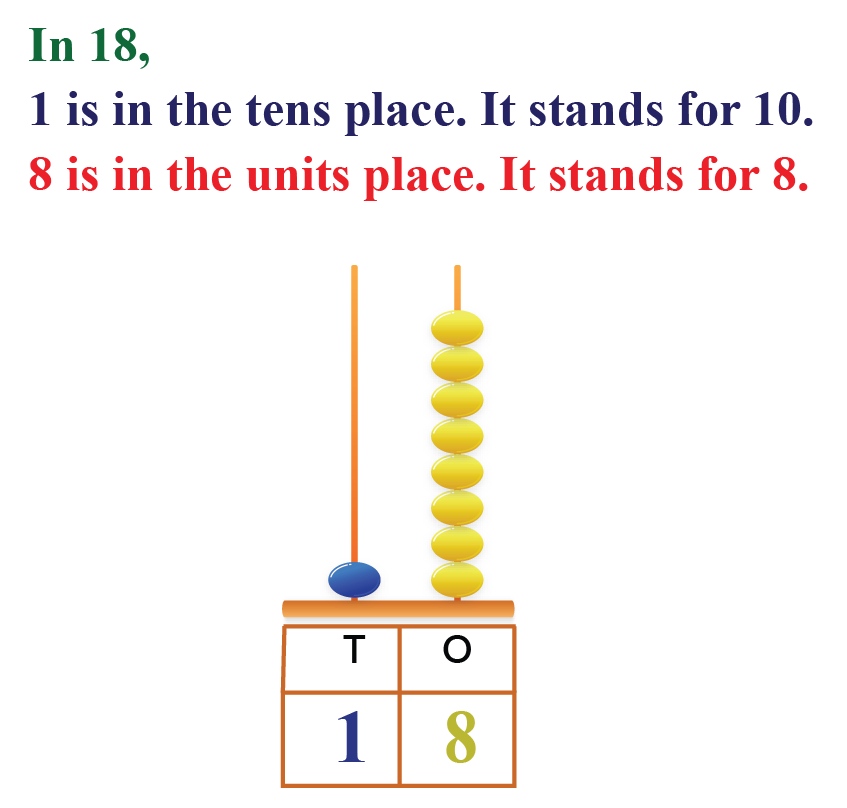### Some Common 2–Digit Numbers

Let us see if you know the answers to the following 5 questions:

1. How many numbers does a dozen have?
2. What is considered an unlucky number?
3. What is a score?
4. How many yards long is a cricket pitch?
5. What number is a quarter century?

The answers to the above questions in order are: $$12, 13, 20, 22$$ and $$25.$$ All these five numbers are 2-digit numbers.

Have a doubt that you want to clear? Get it clarified with simple solutions on 2 digit numbers from our Math Experts at Cuemath’s LIVE, Personalised and Interactive Online Classes.

Make your kid a Math Expert, Book a FREE trial class today!

## Two Digit Number

There are 90 two-digit numbers starting from 10-99

Here is the list of all 2-digit numbers from 10-99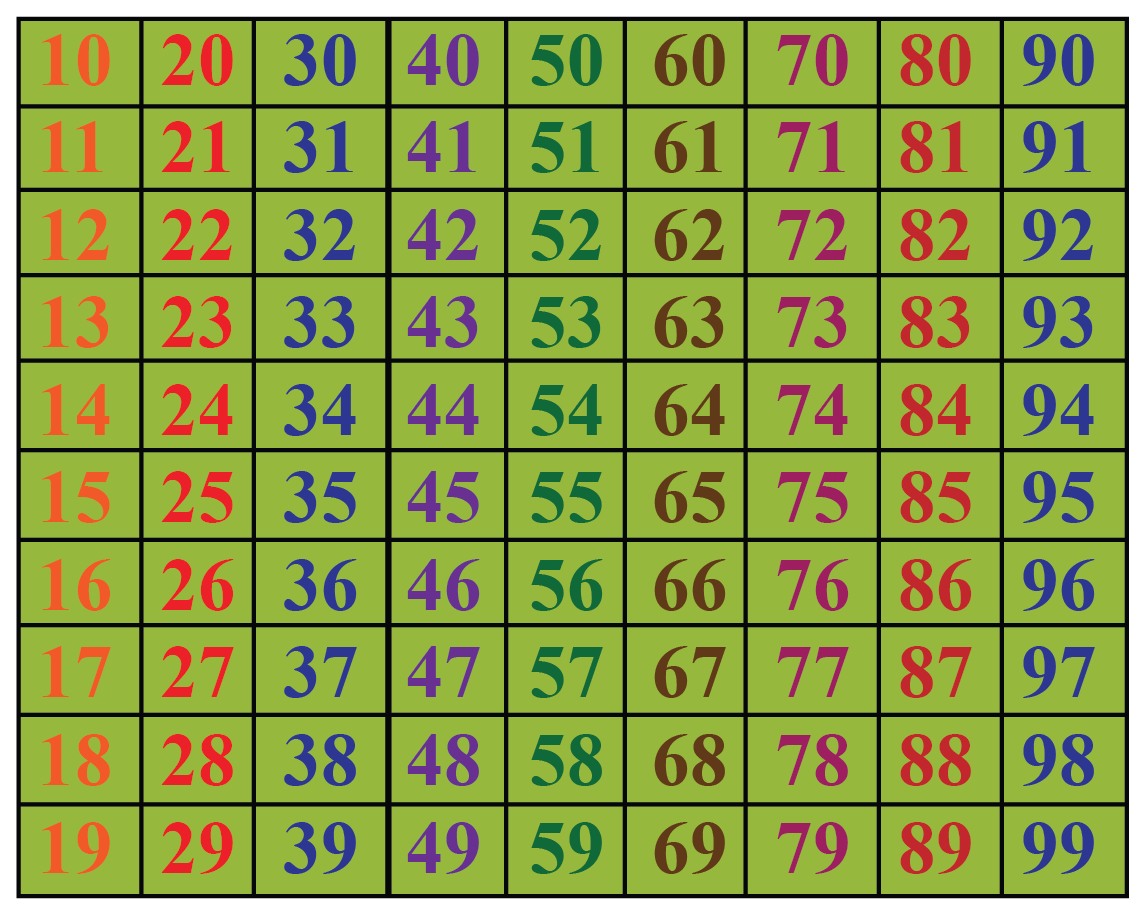## How to Find Number of Digits in a Number?

We saw how we can use our hand to count numbers from 1 to 10

We can also use counters, blocks and rods to count and frame numbers.

A 1-digit number will have only one digit which is between 0-9

A 2-digit number will have two numbers, one in the Tens place and another number in the Ones place.

Example: 25 is a 2-digit number with 2 in the Tens place and 5 in the Ones place.

Similarly, a 3-digit number will have 3 numbers.

Example: 134 is a 3-digit number with 1 in the Hundreds place, 3 in the Tens place and 4 in the Units place.

Can you tell how many digits will be there in a 5-digit number?

## How is it Important?

The Significance (or otherwise) of Zero in 2 digit numbers

We already know that a 2-digit number can be formed by combinations of two of the 10 digits from 0 to 10

But what about those numbers which have 0 in the ten’s place value?

Let us consider the number that would be formed by zero in ten’s place and five in unit’s place.

The number is \begin{align} ( 0 \times 10 ) + ( 5 \times 1 ) = 0 + 5 = 5 \end{align}

Tens digit cannot be 0 because it actually becomes a single digit number.

But that is not the case when the zero is in the unit’s place.

Let us try exchanging the numbers this time, and take five in ten’s place and zero in unit’s place.

The number is \begin{align} ( 5 \times 10 ) + ( 0 \times 1 ) = 50 + 0 = 50 \end{align}

So when it comes to zero, there are nine options which have zero in ten’s place when the number formed is a single digit number, irrespective of which number is in the unit’s place.

Help children see numbers as flexible quantities.

A useful tip is to ask students to break numbers in many different ways.

Eg. 15 is 10 and 5 more.
At the same time, 15 is also 14 and 1 more.
It is also 11 and 4 more.

Play this game often with different numbers.
Start with small numbers like 15 and 20 and then move to larger numbers like 32 and 46

Help children become very comfortable with skip counting by 10
They should be able to skip count by 10 from any number.
Start with the series 10, 20, 30,... but then build comfort by getting students to skip count by 10 from any other starting point.
Eg. from 23: 23, 33, 43, 53,...

List numbers in 10s in one row and one in the second row.
Show the patterns of how numbers from 11 to 99 can be constructed by combining the tens and ones.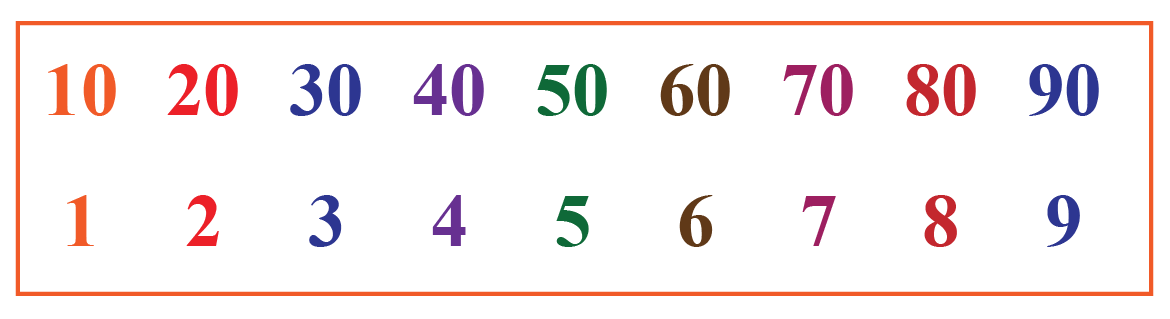Challenging Questions
1.  I am a 2 digit even number more than 6 + 6 and less than 15. Find me!
2. I am a 2 digit number between 50 and 60 and I have my units digit as the number of sides in a square.
3. I am a 2 digit even number greater than 80 and less than 90 and the sum of my digits is 12. Find me!

## Common Mistakes or Misconceptions

• Children can often recite all numbers till 99 (or 100) but may make mistakes when asked to find a larger number between two numbers.
Students sometimes recite numbers from 1 to 100 like they have memorised the letters of the alphabet.
If this happens, then finding the greater number of 14 and 15 is as puzzling as finding the greater letter between C and D.
Children must realise that unlike letters, numbers have a relationship with each other.
15 is exactly one more than 14
For this use number lines, colour counters to associate the sound with the quantity.

• Children tend to make mistakes working with numbers when the tens place value changes.
E.g. when going from 19 to 20, 29 to 30, 39 to 40, etc.
Often 2-digit numbers are introduced without reference to place values.
While this makes it easier to memorise numbers, it may not be the best strategy as children don’t see numbers as constructed from a common set of digits - 0 to 9
Best to use an abacus to model numbers and use Base-10 blocks to help them visualise numbers.

• Children make errors when spelling numbers.
Especially from eleven to nineteen.
There is no substitute to practise.
Children must practise writing number names.
Generally, with a little bit of deliberate and targeted practice, this error can be fixed easily.

Help your child score higher with Cuemath’s proprietary FREE Diagnostic Test. Get access to detailed reports, customised learning plans and a FREE counselling session. Attempt the test now.

## Solved Examples

 Example 1

Ryan said, “There are 6 digits in the number 100000.” Can you count the number of digits in the number and confirm if he is right?

Solution:

On counting the number of digits in 100000, you can observe that there are 5 zeros and 1 one in the number.

Thus, it is a 6-digit number.

 $$\therefore$$  Ryan is correct
 Example 2

A number consists of two digits.

7 in the tens place and 5 in the ones place.

What is this number and write its number name?

Solution:

Let us place the numbers in the place value chart.

Tens place Units Place
7

5

The number is 75 or seventy five.

 $$\therefore$$ The number is 75
 Example 3

Identify the 2-digit numbers represented by the blocks and rods shown below.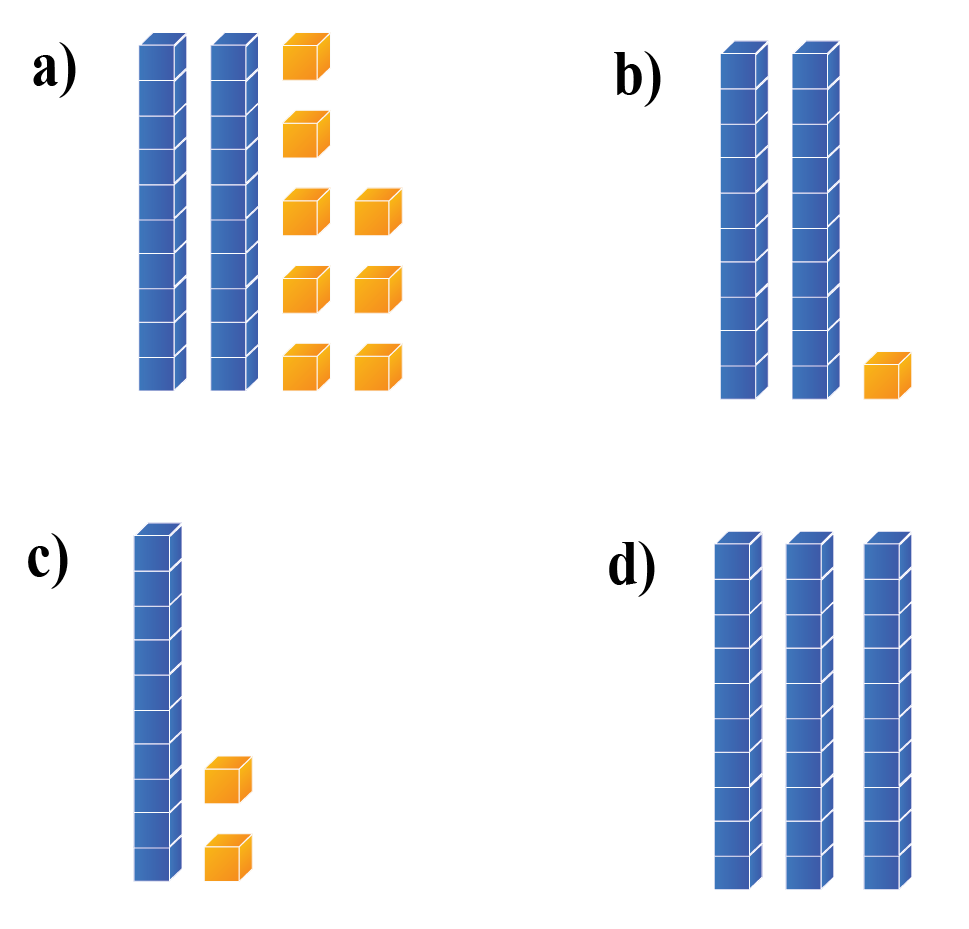Solution:

The blue rods represent the Tens place and the yellow blocks represent the Units place.

S.No Tens place Units Place Number
a) 2

8

28
b) 2 1 21
c) 1 2 12
d) 3 0 30
 $$\therefore$$ The numbers are a) 28 b) 21 c) 12 and d) 30
 Example 4

Find the sum of the largest 2-digit number and the smallest 2-digit number.

Find the number of digits in the number thus found

Solution:

The largest 2-digit number is 99 and the smallest 2-digit number is 10

Their sum is $$99 + 10 = 109$$

109 has 3 digits, it is a 3-digit number.

 $$\therefore$$ the sum is 109 and a 3-digit number
 Example 5

What is the predecessor and successor of the difference of the largest 2-digit number and the number 20?

Solution:

Largest 2-digit number = 99

Difference of 99 and 20 is $$99-20 = 79$$

The predecessor of 79 is 78 and successor of 79 is 80

 $$\therefore$$  The predecessor is 78 and successor is 80
 Example 6

Find the sum of the numbers shown in the abacus.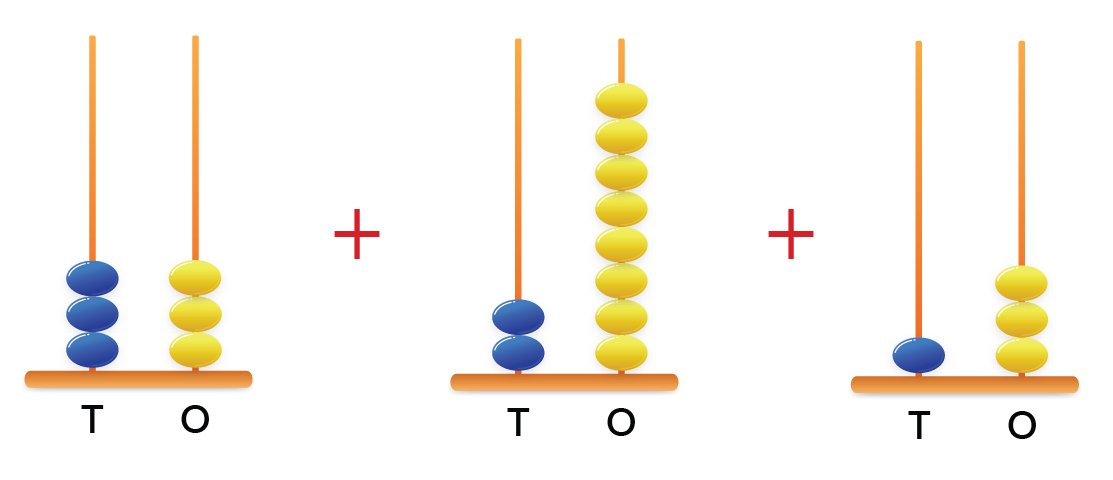Solution:

Let us first identify the numbers, and then add them.

S.No Tens place Units Place Number
1 3

3

33
2 2 8 28
3 1 3  13
Sum    74
 $$\therefore$$ the sum is 74

## Practice Questions

Here are a few activities for you to practice.

IMO (International Maths Olympiad) is a competitive exam in Mathematics conducted annually for school students. It encourages children to develop their math solving skills from a competition perspective.

## 1. How many two-digit numbers are there?

There are 90 two-digit numbers in all.

## 2. How do you add 2-digit numbers?

1. We add the units place of the first number to the units place of the second number.
2. Add the tens place of the 1st number to the tens place of the second number.
3. If there is a carry-over in step - 1 add it to the tens place.

\begin{align} & 29 \\ +& 34 \\ &---\\ =\:&6 3 \\ &--- \end{align}

## 3. What is the largest 2-digit number?

The largest 2-digit number is 99.

## 4. What is the first two-digit number?

The first two-digit number is 10.

Introducing Numbers up to 20
Kg | Worksheet 1
Introducing Numbers up to 20
Kg | Worksheet 2
Consolidating Numbers up to 20
Kg | Worksheet 3
Consolidating Numbers up to 20
Kg | Worksheet 4

More Important Topics
Numbers
Algebra
Geometry
Measurement
Money
Data
Trigonometry
Calculus